17 Sep

It’s high time that S∞ got back to its core: mathematical constructions you can build. Here’s an attractive star-shaped polyhedron made with a weaving technique that I am indebted to Jürgen Richter-Gebert for introducing me to. It’s called the “small stellated dodecahedron,” and is one of the Kepler-Poinsot regular polyhedra. (Unlike the usual five Platonic solids, its edges intersect each other at points besides the twelve vertices.) We’ll call it the “SSD” for short.

MaterialsTools
30 equal-length rodsScissors
32 rubber bands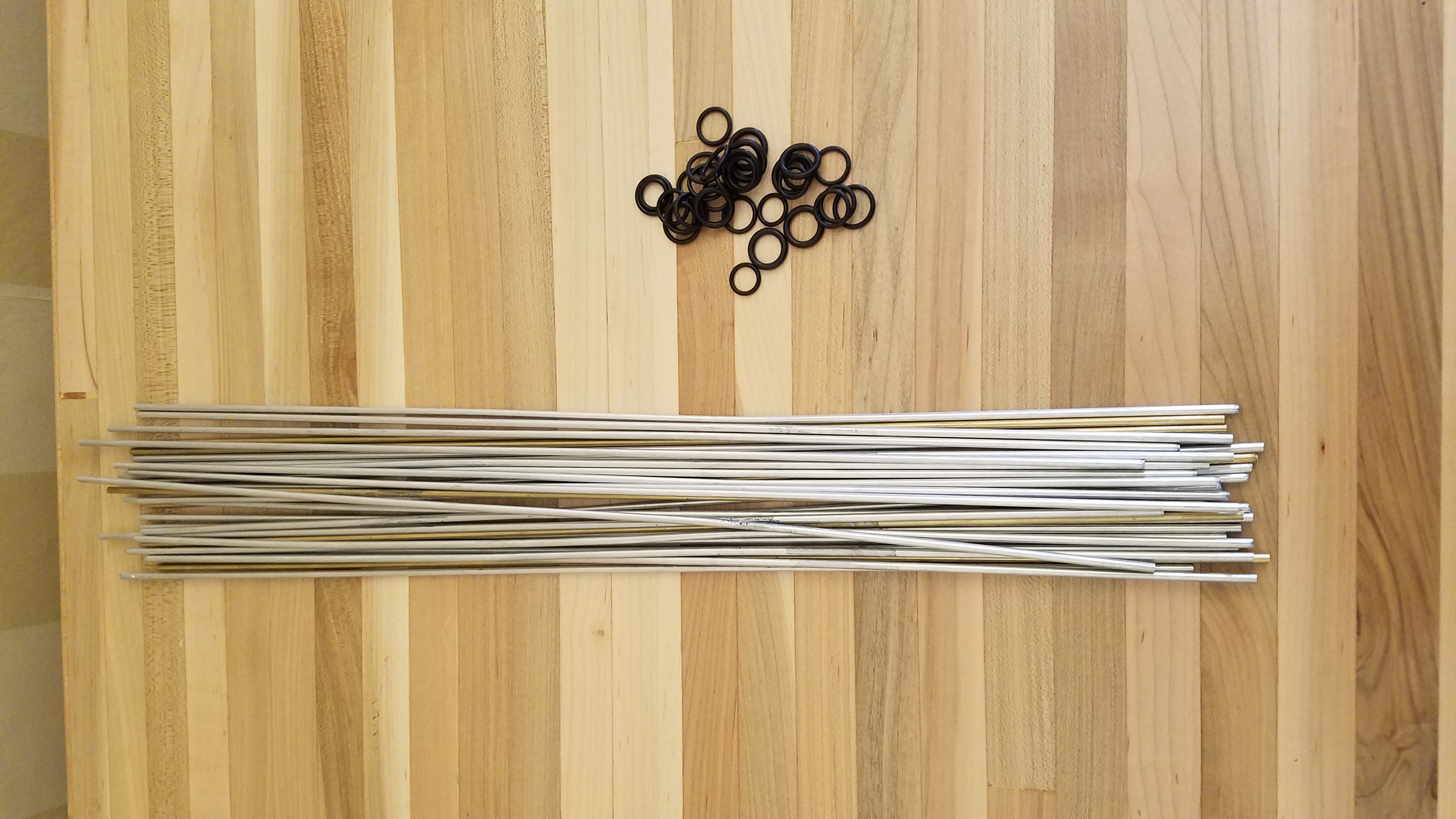To build this, you first need 30 rods all the same length, made of some material that has a bit of give (hint: not glass rods). You can choose almost any material; here, I’ve used mostly aluminum tubes, with five contrasting brass tubes of the same diameter to highlight the first star you make. Possibilities include thin dowels, bamboo strips, pipe cleaners, and more.

You also need 32 rubber bands. They should be sized so that it’s easy enough to get them around five rods simultaneously, but then they should hold that thickness of material securely (of course you can double or triple up the bands if need be). You can use almost any type of elastic bands: ordinary office rubber bands, colored hair elastics, or rubber O-rings as I’ve used here.

Before you get started assembling the SSD, you should mark the locations that the rubber bands will go on each rod. To find these locations, first pick an amount of each rod that will extend past each vertex for security of the connections. For example, I used 18-inch rods, so I chose that the rods would extend 1/2 inch past each vertex. If you are making a larger version, you’d want to pick something longer; if you are making a smaller SSD, I wouldn’t go much below 1/2 inch because the vertex connections might start slipping apart too easily.

Subtract twice the amount you are leaving at the vertices from the length of the rods to get the distance between the vertices. In my case, that’s 18 – 2×(1/2) = 17 inches. Now multiply this value by 2/(3+√5); for 17 inches, this gives about 6.49 inches. (Hopefully I will get a chance to write a MathStream post that explains this calculation.) Finally add back in the amount you’re leaving at the vertices; this step gives me 6.99 inches, which we should of course round to 7 inches. That’s the distance you want to mark on the rods from each end (two marks per rod). With my 18-inch rods, I just lay them next to a ruler and mark at 7 and 11 inches.

Ok, now all is ready for construction. The basic step you’ll be doing over and over is placing two rods side-by-side and putting a rubber band around both of them and sliding it down to the first mark on the rods.

We’ll call this basic unit a “linked pair.” Take two linked pairs and put them side by side with their connections on the same side, and put a third rubber band around one rod from each pair at the ends opposite the existing connections. Slide the rubber band down so that you have a zig-zag chain of four rods.

Connect a fifth rod to one at either side, continuing the zig-zag.

Now connect the free end of this fifth rod to the free end of the first rod of the zig-zag, as shown on the right.

This is a configuration that you can now wiggle around until it forms a five-pointed star, as shown to the left. Important: looking at the two junctions on each rod, the rod must be underneath at the first junction and on top at the second junction as you proceed clockwise around the inner pentagon. Make sure that every one of the five rods follows this pattern before continuing, It’s this under-over, under-over pattern that constitutes the weaving in this construction, and that same under-over, under-over will continue throughout the entire construction.

Next, at each of the points of the star you’re forming, you can slip one rod under the other to continue the under-over junction pattern of the rods. These points of the star will eventually be vertices of the SSD.

Add a rubber band to secure each of the points, and congratulations, you’ve just made your first star out of 12.

To continue toward the SSD, take five rods and line them all up and put a rubber band around all five of them and slide it down just a short way, roughly the amount you decided to leave at the vertices above. You should then be able to splay the rods out so that the far ends are at the points of a pentagon, and there is a little clockwise swirl of the near ends of the rods (see the picture to the right).

Place each of the far ends just counterclockwise of one of the internal junctions of the star you made, in order around the star (as shown on the left).

Insert each of the far ends into the rubber band at the corresponding junction, making sure to keep it within the triangle of the star that it started in.

Now push all of the five new rods through those junctions until the second mark reaches the junction. That completes the first full vertex of your SSD.

If you look at your structure in progress from the side, you will see three out of five sides of another star. We want to complete that to a full star. So take a linked pair, and link one free end of the pair to each of the two sides of the star sticking out. Push those links in a little way and make sure the junctions all continue the under-over pattern of the first star. If all looks well, you can slide all of the connections until they reach the marks.

To complete the second star, slip the points of the star past each other as you did with the first star, and secure with rubber bands (there will already be rubber bands for this in place at two of the vertices).

Now rotate the structure 1/5 of a turn around the original star. You’ll see another star with only three sides in place. You want to complete this in a similar fashion. The only difference is that this time, you only make one entirely new link; at the other end of the linked pair you’re adding, you feed the rod through the existing rubber band at an internal junction. Make sure to continue the same under-over pattern throughout. The new rod should pass through a triangle in the second star that does not yet have a rod passing through it (it should not pass outside the second star or through the pentagon of the second star).

When that one’s done, add two more linked pairs to complete two more stars in exactly the same fashion.

The next linked pair is very similar to these; you just have to feed both of its free ends through existing junctions. When that’s done, you’ve finished six of the twelve stars in the SSD.

To continue from here, take a single rod (not a linked pair) and feed it through any of the partial vertices and the nearest internal junction that only has two rods at it, as shown.

Adding that rod will create a new partial star with three edges in place. Complete that one just the way you did the third star.

You’re getting close now. Complete two more stars like the third, and then one like the sixth.

That leaves just a single line of four junctions that does not have a rod going through them (shown down the middle of the image above). Carefully insert the final rod through all four junctions – it’s probably easiest to pass it through the two internal ones first, then tuck the two ends in at the vertices, to produce something like this. (This last edge is again down the middle of the image below.)

Now comes the exciting final part: cut away all of the rubber bands at the internal junctions. They were just there to stabilize things during construction; they’re not actually needed to hold the SSD together once it’s built, thanks to all of that weaving that we did. And voila!

In fact, if you used a high-friction material like bamboo strips or pipe cleaners, it’s often possible to cut away the rubber bands at the vertices as well. I don’t have a picture of this, as the metal rods are too slippery for the vertices to hold. If you want to try, just proceed carefully, and if any of the vertex junctions “pop,” tie it back up immediately and don’t cut any more (unless you want to quickly revert to a pile of separate rods).

If you like this construction and happen to be in Providence on 2019 Sep 28, come to the ICERM Open House at WaterFire Providence and help build a glowing version with 10-foot pipes for the rods!

30 Jul

If you’ve come here as a result of a puzzling postcard you may have come across, welcome to Studio Infinity! We hope you’ll enjoy looking at some of the other content below as well, but here are the three posts corresponding to the problems you can find on those postcards, each of which links to a solution.

And, as I’ve mentioned here before, I invite you all to submit a problem or solution to Math Horizons Playground.

30 Jul

How does the value of the following improper integral compare to 1? I.e., is it smaller, larger, or exactly equal to 1?

(This problem was proposed to Math Horizons Playground by Mehtaab Sawney of Commack High School. And for all of you $\pi$-ists out there, $\tau$ is of course just the radian measure of a full circle, i.e., $\tau=2\pi$.)

Stumped? You can peek at the solution using the password “strapyb”.

30 Jul

30 Jul

For definiteness, the unlabeled points do in fact trisect each of the sides of the triangle.

30 Jul

30 Jul

What are the next few terms in this sequence of smallish numbers?

# 1 1 13131 131 131 131 1 1321 132131 131 1 1321 1321 13 ? ? ?

(And, of course, what’s the rule generating the sequence?)

Stumped? You can peek at the solution using the password “1p1a2s”.

30 Jul

21 Oct

As one of their projects, students in my class this fall wrote about something they’ve seen that sparked mathematical questions and ideas for them. The series of posts below shows the results of their work. I hope you’ll enjoy seeing math through their eyes as much as I have.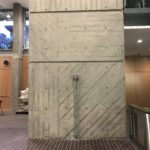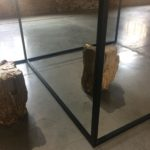24 Jul

I recently purchased a large number of styrofoam balls as supplies for an upcoming build (about which I will post later). The plans for that build required the diameter of the styrofoam balls, to pretty high accuracy. Although the balls were nominally 4 1/2 inches in diameter, I had noticed in a craft shop that their products also had diameters listed in whole numbers of millimeters that were close to the inch ratings, but not precisely equal. Not only could I not find the millimeter diameters listed for this particular size, I needed to know which was closer to reality, the U.S. or the metric measurement. (I had a hunch that given the world-wide nature of manufacturing and the fact that only the U.S. does not use metric, the metric measurements were more likely to be accurate.)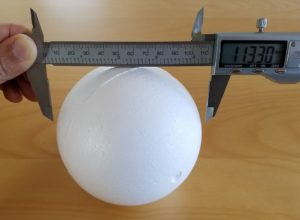Unfortunately, my calipers did not have long enough jaws to clamp down onto the styrofoam balls, as you can see above. So how could I accurately measure the diameter of the sphere? You can’t exactly stick a ruler through the ball, and even if you could, how would you locate two diametrically opposed points? There’s a nice trick with a circle, in which you pick any two points, and then draw a line through one of them perpendicular to the line between them. Where that line intersects the circle is diametrically opposite the other point. (This works because the hypotenuse of any right triangle inscribed in a circle is a diameter.) But is there a three-dimensional version of this?

Fortunately, I was not the first person to face this conundrum; you can find this exact question on Math StackExchange. So first I tried the “accepted” answer: find two planes at exactly right angles with each other (I used a bookshelf, checked for true with a square), shove the sphere into the corner where the planes meet, and measure the distance between the plane and the point of contact to get the radius. Clever, huh?

But in practice, it’s pretty difficult to see exactly where the sphere touches the shelf. As you can see in the photo above, it looks consistent with a 57mm radius but it also looked consistent with a 57.5mm or even a 58mm radius. I wanted to double check with a more accurate method.

Fortunately, there was another answer on that page, involving measuring multiple distances on the surface of the sphere (easy to do with calipers), followed by a rather lengthy and involved calculation. But a little experimentation and figuring arrived at the following method, which is pretty quick and simple, so I thought it deserved its own post.

First,

Materials
sphere to measure
compass
calipers
pins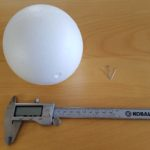pick a convenient, round distance that appears to be in the neighborhood of a third to a quarter of the way around the sphere; for my styrofoam pieces, I chose 90mm. Using calipers (or a ruler), set the compass to exactly this distance. Choose an arbitrary point on the sphere, and draw a circle with the compass. (You can just see the circle faintly in the picture below.)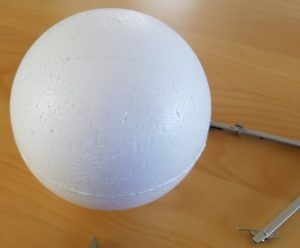Now choose another arbitrary point on this circle. We want to find the two points on the circle at that same chosen distance away from this second point. So put the point of the compass on this second point, and draw two small arcs intersecting the circle, one on either side of the chosen point. Carefully measure the straight-line distance between these two points of intersection (not the distance on the surface of the sphere, i.e., use calipers, not a bendy ruler or measuring tape).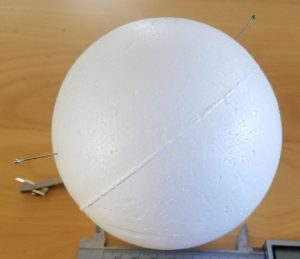I stuck a pin into each of the two points as an aid to positioning the jaws of the caliper precisely at those points, as you can see at left. Call that measured distance F; in my case, I got 101.2mm. Call the original chosen distance (the one you drew the circle and arcs with) A. Then the radius of the sphere is ½A√((4A²-F²)/(3A²-F²)). With my numbers, A = 90 and F = 101.2, this came out numerically to 56.495… Hence, I conclude that the actual diameter of the styrofoam balls is 113mm, just slightly below 4 1/2 inches (numerically it comes out to 4.4488 in).

To see exactly why this works and how the formula is derived, check out the StackExchange page. A very pleasant case of the math actually working out to solve a practical problem!

14 Apr

Hey, I have recently become problem editor for the undergraduate Math Horizons magazine of the Mathematical Association of America. So I’d love if you have problems/mathematical puzzles to submit to the column. The official submission blurb follows, and of course you will be credited in the Magazine.

The Playground features problems for students at the undergraduate and (challenging) high school levels. Problems or solutions (including more elegant or extended solutions to Carousel problems) should be submitted to MHproblems@maa.org or MHsolutions@maa.org, respectively. Paper submissions may be sent to Glen Whitney, ICERM, 121 South Main Street, Box E, 11th Floor, Providence, RI 02903 . Please include your name, email address, and school or institutional affiliation, and indicate if you are a student. If a problem has multiple parts, solutions for individual parts will be accepted. Unless otherwise stated, problems have been solved by their proposers.

11 Apr

This post contains the details of the claim made in “More Spherical Construction” that you can determine the side length of a spherical square from the ratio between the lengths of its diagonals. We’ll do this on a sphere of radius one; everything scales by a factor of the radius for a general sphere.

The advantage of working on a sphere of radius one is that then the side length is nothing other than the measure of the central angle (in radians) between two adjacent vertices of the square. Similarly, the diagonal is the central angle between opposite vertices of the square.

The easiest way to construct a square on the unit sphere is to place its center at the intersection of the sphere and the x-axis, and then choose a (positive) angle $\theta \leq \tau/4$ (where $\tau = 2\pi$ is the radian measure of a full circle) and place two vertices at plus or minus $\theta$ (from the square’s center) in the longitudinal direction and two vertices at plus or minus $\theta$ in the latitudinal direction. In spherical coordinates, the center is at $(1,0,\tau/4)$ and the four vertices are at $(1,-\theta,\tau/4), (1,\theta,\tau/4), (1,0,\tau/4-\theta), (1,0,\tau/4+\theta)$. For this square, the diagonal is obviously $2\theta$; it remains only to compute the side length of the square.

Using the great-circle distance formula on the two points $(1,\theta,\tau/4)$ and $(1,0,\tau/4-\theta)$ yields a side length of $\arccos(\cos(\tau/4)\cos(\tau/4-\theta) + \sin(\tau/4)\sin(\tau/4-\theta)\cos(\theta)\;).$

Since $\cos(\tau/4)$ is 0 and $\sin(\tau/4)$ is 1, this expression equals $\arccos(\sin(\tau/4-\theta)\cos(\theta)).$

And further, $\sin(\tau/4-\theta) = \cos(\theta)$, so the side length is just $\arccos(\cos^2 \theta).$

Therefore, the ratio of the diagonal to the side of the square is $2\theta/\arccos(\cos^2 \theta)$. Graphing this on the allowed interval $(0,\tau/4]$ for $\theta$ immediately demonstrates the claims made in the referring post: no value of the ratio is repeated for different values of $\theta$, so the ratio determines the diagonal (and side length) of the square; the maximum value is 2; and any value greater than $\sqrt2$ is achievable.

If you’d rather not rely on graphing software to extract these facts, but rather demonstrate them just from the formulas, here’s how you can proceed. The value 2 at $\theta=\tau/4$ comes from straight substitution. The value at 0 by substitution is the indeterminate 0/0, so we obtain the value by L’Hôpital’s rule: the derivative of the numerator is 2, but the derivative of the denominator is $\frac{2\cos \theta}{\sqrt{1+\cos^2\theta}},$ so the diagonal-side ratio has the limiting value $\sqrt2$ at $\theta =0$. Finally, we need to show that the ratio is monotone increasing on the interval of interest. By the quotient rule, this is equivalent to showing that on this interval $\arccos(\cos^2\theta) > \frac{2\theta\cos\theta}{\sqrt{1+\cos^2\theta}}.$ Both sides are 0 at $\theta=0$, so we can take derivatives one more time; the derivative of the left-hand side is of course $2\cos\theta(1+\cos^2\theta)^{-1/2}$ again, which on this interval is always larger than the derivative of the right-hand side, namely $2\cos\theta(1+\cos^2\theta)^{-1/2} – 2\theta\sin\theta(1+\cos^2\theta)^{-3/2}$. So the left-hand side is also always larger than the right hand side, i.e., the derivative of the diagonal-side ratio is positive on this interval.